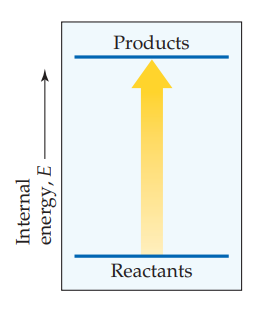×
Get Full Access to Chemistry: The Central Science - 14 Edition - Chapter 5 - Problem 5.3
Get Full Access to Chemistry: The Central Science - 14 Edition - Chapter 5 - Problem 5.3

×ISBN: 9780134414232 1274

## Solution for problem 5.3 Chapter 5

Chemistry: The Central Science | 14th Edition

• Textbook Solutions
• 2901 Step-by-step solutions solved by professors and subject experts
• Get 24/7 help from StudySoup virtual teaching assistantsChemistry: The Central Science | 14th Edition

4 5 1 284 Reviews
27
4
Problem 5.3

Consider the accompanying energy diagram.

(a) Does this diagram represent an increase or decrease in the internal energy of the system?

(b) What sign is given to $$\Delta E$$ for this process?

(c) If there is no work associated with the process, is it exothermic or endothermic? [Section 5.2]Text Transcription:

\Delta E

Step-by-Step Solution:
Step 1 of 3

Week 10 Notes Buffers ● Buffer = when a weak acid and its conjugate weak base are both present ● Buffer region = beginning of titration up until pH spikes ● Henderson-Hasselbalch: super useful equation ○ pH = -log(pKa) + log[Base]/[Acid] ■ Any units can be used for acid and base, like M, grams, etc. ● ½ equivalence point: pH = pKa Titrations ● Types of Titrations ○ Strong acid strong base: pH = 7 at equivalence point ○ Strong acid weak base: pH < 7 at equivalence point ○ Weak acid strong base: pH > 7 at equivalence point ● Indicators ○ pKa of acid should be in the region where indicator changes color ○ Indicator should change color during the spike in pH on graph/ around the equivalence point ● At equivalence point/ end point of the titration, [H+] = [OH-] Solubility ● The lower the Ksp value, the sooner the substance will precipitate out of solution -17​ -12 ○ ex) AgI: Ksp = 2.6 x 10​ , PbI​ :2​sp = 3.8 x 10​ ■ AgI will precipitate first ● If Q < Ksp, reaction will proceed spontaneously ● Concentration of the original solid does NOT affect solubility, but a common ion already in solution - ​ ○ ex) take AgI (s) . If there are already I​ ions in solution, this will decrease the solubility because the reaction will shift to t

Step 2 of 3

Step 3 of 3

## Discover and learn what students are asking

Calculus: Early Transcendental Functions : Exponential and Logarithmic Functions
?Evaluating an Expression In Exercises 1 and 2, evaluate the expressions. (a) $$64^{1 / 3}$$ (b) $$5^{-4}$$ (c) \(\left(\fra

Calculus: Early Transcendental Functions : Integration by Tables and Other Integration Techniques
?In Exercises 47-52, verify the integration formula. \(\int \frac{1}{\left(u^{2} \pm a^{2}\right)^{3 / 2}} d u=\frac{\pm u}{a^{2} \sqrt{u^{2}

Statistics: Informed Decisions Using Data : An Overview of Nonparametric Statistics
?Describe the difference between parametric statistical procedures and nonparametric statistical procedures.

Unlock Textbook Solution